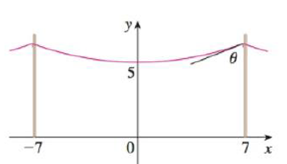Chapter 3.11, Problem 51E### Single Variable Calculus: Early Tr...

8th Edition
James Stewart
ISBN: 9781305270343

#### Solutions

Chapter
Section### Single Variable Calculus: Early Tr...

8th Edition
James Stewart
ISBN: 9781305270343
Textbook Problem

# A telephone line hangs between two poles 14 m apart in the shape of the catenary y = 20 cosh(x/20) – 15, where x and y are measured in meters.(a) Find the slope of this curve where it meets the right pole.(b) Find the angle θ between the line and the pole.(a)

To determine

To find: The slope of the given curve where it meets the right pole.

Explanation

Given:

The distance between two poles is 14 m,

The telephone line is hangs between two poles,

The shape of the catenary is y=20cosh(x20)15.

Formula used:

The hyperbolic functions ddx(coshx)=sinhx.

Calculation:

From given graph, it is observed that the right pole located at 7 in x axis.

Obtain the derivative of y=20cosh(x20)15 at x = 7.

dydx=ddx(20cosh(x20)15)=ddx(20cosh(x20))ddx(15)=20ddx(cosh(x20))0=20ddx(cosh

(b)

To determine

To find: The angle θ between line and pole.

### Still sussing out bartleby?

Check out a sample textbook solution.

See a sample solution

#### The Solution to Your Study Problems

Bartleby provides explanations to thousands of textbook problems written by our experts, many with advanced degrees!

Get Started

#### In problems 45-62, perform the indicated operations and simplify. 53.

Mathematical Applications for the Management, Life, and Social Sciences

#### Which is the graph of ?

Study Guide for Stewart's Single Variable Calculus: Early Transcendentals, 8th

#### For

Study Guide for Stewart's Multivariable Calculus, 8th